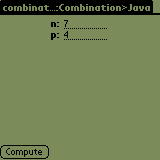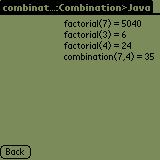Home > Palm > Samples > Combination > JavaPalm sample in Java

####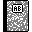Purpose

This is the J2ME version of our combination application. It's one of the Palm Samples.

####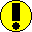Requirements

You need J2ME (see tools section).

####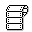Source code

```/*
* Combination.java
* J2ME
* Java
*/

package samples;

import javax.microedition.midlet.*;
import javax.microedition.lcdui.*;

/**
* A sample that computes factorial(n) and combination(n,p)
*/
public class Combination extends MIDlet implements CommandListener {
private Display display;
private Form frmIn, frmOut;
private TextField   fieldN, fieldP;
private Command exitCommand = new Command("Exit", Command.EXIT, 1);
private Command computeCommand = new Command("Compute", Command.OK, 1);
private Command backCommand = new Command("Back", Command.BACK, 1);

/*
* Construct a new Combination.
*/
public Combination() {
display = Display.getDisplay(this);
}

/**
* Construct and display UI
*/
public void startApp() {
frmIn = new Form("Combination>Java");

frmIn.append(fieldN = new TextField("n: ", "", 4, TextField.NUMERIC));
frmIn.append(fieldP = new TextField("p: ", "", 4, TextField.NUMERIC));

frmIn.setCommandListener(this);

display.setCurrent(frmIn);
}

public void commandAction(Command c, Displayable s) {
if (c == computeCommand) {
int n = java.lang.Integer.parseInt(fieldN.getString()),
p = java.lang.Integer.parseInt(fieldP.getString());
go(n, p);
Display.getDisplay(this).setCurrent(frmOut);
}
else
if (c == backCommand) {
Display.getDisplay(this).setCurrent(frmIn);
frmOut = null;
}
else
if (c == exitCommand) {
destroyApp(false);
notifyDestroyed();
}
}

/**
* Time to pause, free any space we don't need right now.
*/
public void pauseApp() {
display.setCurrent(null);
frmIn = frmOut = null;
fieldN = fieldP = null;
}

/**
* Destroy must cleanup everything.
*/
public void destroyApp(boolean unconditional) {
}

public long factorial(int n) {
return (n <= 1) ? 1 : n*factorial(n-1); // he-he... let's mix a ternary operator (?) and recursion...
}

public long combination(int n, int p) {
return factorial(n) / (factorial(n - p) * factorial(p));
}

public void go(int n, int p) {
frmOut = new Form("Combination>Java");

frmOut.append("factorial(" + n + ") = " + factorial(n) + "\n");
frmOut.append("factorial(" + (n-p) + ") = " + factorial(n-p) + "\n");
frmOut.append("factorial(" + p + ") = " + factorial(p) + "\n");
frmOut.append("combination(" + n + "," + p + ") = " + combination(n, p) + "\n");

frmOut.setCommandListener(this);
}
}
```

####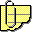Comments

We'll only import a minimal set of J2ME classes. Just enough for our simple UI. Please note that this J2ME version of combination also runs on a Java phone as is. Yes, you read correctly: you don't have to change any line of code! You'll find the .prc in the ZIP file mentioned above. If you'd like to deploy this app to a Java phone, you'll need to use a specific tool to package it and upload it on the device.

The UI is created in startApp(), events are handled in commandAction(Command c, Displayable s).

The core functions are located at the end of the .java file: factorial(), combination() and go(). Here, note that the overhead is pretty limited, compared with the Palm SDK version.